# 19 Results

View
Selected filters:
• MCCRS.Math.Content.6.EE.A.3Only Sharing Permitted
CC BY-NC-ND
Rating

This lesson unit is intended to help teachers assess how well students are able to visualize two-dimensional cross-sections of representations of three-dimensional objects. In particular, the lesson will help you identify and help students who have difficulties recognizing and drawing two-dimensional cross-sections at different points along a plane of a representation of a three-dimensional object.

Subject:
Geometry
Material Type:
Assessment
Lesson Plan
Provider:
Shell Center for Mathematical Education
U.C. Berkeley
Provider Set:
Mathematics Assessment Project (MAP)
04/26/2013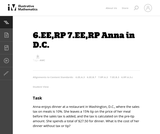Unrestricted Use
CC BY
Rating

This is a task from the Illustrative Mathematics website that is one part of a complete illustration of the standard to which it is aligned. Each task has at least one solution and some commentary that addresses important asects of the task and its potential use. Here are the first few lines of the commentary for this task: Anna enjoys dinner at a restaurant in Washington, D.C., where the sales tax on meals is 10%. She leaves a 15% tip on the price of her meal before the s...

Subject:
Mathematics
Material Type:
Activity/Lab
Provider:
Illustrative Mathematics
Provider Set:
Illustrative Mathematics
Author:
Illustrative Mathematics
03/17/2013Unrestricted Use
CC BY
Rating

This module contains the an algebraic expressions and equations from Elementary Algebra by Denny Burzynski and Wade Ellis, Jr.

Subject:
Algebra
Material Type:
Provider:
Rice University
Provider Set:
Connexions
Author:
Denny Burzynski
02/16/2011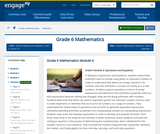Conditional Remix & Share Permitted
CC BY-NC-SA
Rating

In Module 4, Expressions and Equations, students extend their arithmetic work to include using letters to represent numbers in order to understand that letters are simply "stand-ins" for numbers and that arithmetic is carried out exactly as it is with numbers. Students explore operations in terms of verbal expressions and determine that arithmetic properties hold true with expressions because nothing has changedthey are still doing arithmetic with numbers. Students determine that letters are used to represent specific but unknown numbers and are used to make statements or identities that are true for all numbers or a range of numbers. They understand the relationships of operations and use them to generate equivalent expressions, ultimately extending arithmetic properties from manipulating numbers to manipulating expressions. Students read, write and evaluate expressions in order to develop and evaluate formulas. From there, they move to the study of true and false number sentences, where students conclude that solving an equation is the process of determining the number(s) that, when substituted for the variable, result in a true sentence. They conclude the module using arithmetic properties, identities, bar models, and finally algebra to solve one-step, two-step, and multi-step equations.

Subject:
Numbers and Operations
Material Type:
Module
Provider:
New York State Education Department
Provider Set:
EngageNY
12/17/2013Only Sharing Permitted
CC BY-NC-ND
Rating

This lesson unit is intended to help you assess how well students are able to: Perform arithmetic operations, including those involving whole-number exponents, recognizing and applying the conventional order of operations; Write and evaluate numerical expressions from diagrammatic representations and be able to identify equivalent expressions; apply the distributive and commutative properties appropriately; and use the method for finding areas of compound rectangles.

Subject:
Geometry
Material Type:
Assessment
Lesson Plan
Provider:
Shell Center for Mathematical Education
U.C. Berkeley
Provider Set:
Mathematics Assessment Project (MAP)
04/26/2013Conditional Remix & Share Permitted
CC BY-NC
Rating

Subject:
Mathematics
Material Type:
Full Course
Provider:
Pearson
10/06/2016Rating

Expressions

Type of Unit: Concept

Prior Knowledge

Students should be able to:

Write and evaluate simple expressions that record calculations with numbers.
Use parentheses, brackets, or braces in numerical expressions and evaluate expressions with these symbols.
Interpret numerical expressions without evaluating them.

Lesson Flow

Students learn to write and evaluate numerical expressions involving the four basic arithmetic operations and whole-number exponents. In specific contexts, they create and interpret numerical expressions and evaluate them. Then students move on to algebraic expressions, in which letters stand for numbers. In specific contexts, students simplify algebraic expressions and evaluate them for given values of the variables. Students learn about and use the vocabulary of algebraic expressions. Then they identify equivalent expressions and apply properties of operations, such as the distributive property, to generate equivalent expressions. Finally, students use geometric models to explore greatest common factors and least common multiples.

Subject:
Mathematics
Algebra
Material Type:
Unit of Study
Provider:
PearsonConditional Remix & Share Permitted
CC BY-NC
Rating

Students use a rectangular area model to understand the distributive property. They watch a video to find how to express the area of a rectangle in two different ways. Then they find the area of rectangular garden plots in two ways.Key ConceptsThe distributive property can be used to rewrite an expression as an equivalent expression that is easier to work with. The distributive property states that multiplication distributes over addition.Applying multiplication to quantities that have been combined by addition: a(b + c)Applying multiplication to each quantity individually, and then adding the products together: ab + acThe distributive property can be represented with a geometric model. The area of this rectangle can be found in two ways: a(b + c) or ab + ac. The equality of these two expressions, a(b + c) = ab + ac, is the distributive property.Goals and Learning ObjectivesUse a geometric model to understand the distributive property.Write equivalent expressions using the distributive property.

Subject:
Algebra
Geometry
Material Type:
Lesson Plan
Provider:
Pearson
09/21/2015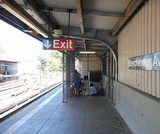Conditional Remix & Share Permitted
CC BY-NC
Rating

Students critique the work of other students and revise their own work based on feedback from the teacher and peers.Key ConceptsConcepts from previous lessons are integrated into this unit task: rewriting expressions, using parentheses, and using the distributive property. Students apply their knowledge, review their work, and make revisions based on feedback from you and their peers. This process creates a deeper understanding of the concepts.Goals and Learning ObjectivesApply knowledge of expressions to correct the work of other students.Track and review the choice of strategy when problem solving.

Subject:
Mathematics
Material Type:
Lesson Plan
Provider:
Pearson
09/21/2015Conditional Remix & Share Permitted
CC BY-NC
Rating

Students express the lengths of trains as algebraic expressions and then substitute numbers for letters to find the actual lengths of the trains.Key ConceptsAn algebraic expression can be written to represent a problem situation. More than one algebraic expression may represent the same problem situation. These algebraic expressions have the same value and are equivalent.To evaluate an algebraic expression, a specific value for each variable is substituted in the expression, and then all the calculations are completed using the order of operations to get a single value.Goals and Learning ObjectivesEvaluate expressions for the given values of the variables.

Subject:
Algebra
Material Type:
Lesson Plan
Provider:
Pearson
09/21/2015Conditional Remix & Share Permitted
CC BY-NC
Rating

Students write an expression for the length of a train, using variables to represent the lengths of the different types of cars.Key ConceptsA numerical expression consists of a number or numbers connected by the arithmetic operations of addition, subtraction, multiplication, division, and exponentiation.An algebraic expression uses letters to represent numbers.An algebraic expression can be written to represent a problem situation. Sometimes more than one algebraic expression may represent the same problem situation. These algebraic expressions have the same value and are equivalent.The properties of operations can be used to make long algebraic expressions shorter:The commutative property of addition states that changing the order of the addends does not change the end result:a + b = b + a.The associative property of addition states that changing the grouping of the addends does not change the end result:(a + b) + c = a + (b + c).The distributive property of multiplication over addition states that multiplying a sum by a number gives the same result as multiplying each addend by the number and then adding the products together:a(b + c) = ab + ac.Goals and Learning ObjectivesWrite algebraic expressions that describe lengths of freight trains.Use properties of operations to shorten those expressions.

Subject:
Algebra
Material Type:
Lesson Plan
Provider:
Pearson
09/21/2015Conditional Remix & Share Permitted
CC BY-NC
Rating

Students represent problem situations using expressions and then evaluate the expressions for the given values of the variables.Key ConceptsAn algebraic expression can be written to represent a problem situation.To evaluate an algebraic expression, a specific value for each variable is substituted in the expression, and then all the calculations are completed using the order of operations to get a single value.Goals and Learning ObjectivesDevelop fluency in writing expressions to represent situations and in evaluating the expressions for given values.

Subject:
Algebra
Material Type:
Lesson Plan
Provider:
Pearson
09/21/2015Rating

Getting Started

Type of Unit: Introduction

Prior Knowledge

Students should be able to:

Solve and write numerical equations for whole number addition, subtraction, multiplication, and division problems.
Use parentheses to evaluate numerical expressions.
Identify and use the properties of operations.

Lesson Flow

In this unit, students are introduced to the rituals and routines that build a successful classroom math community and they are introduced to the basic features of the digital course that they will use throughout the year.

An introductory card sort activity matches students with their partner for the week. Then over the course of the week, students learn about the lesson routines: Opening, Work Time, Ways of Thinking, Apply the Learning, Summary of the Math, and Reflection. Students learn how to present their work to the class, the importance of taking responsibility for their own learning, and how to effectively participate in the classroom math community.

Students then work on Gallery problems to further explore the program’s technology resources and tools and learn how to organize their work.

The mathematical work of the unit focuses on numerical expressions, including card sort activities in which students identify equivalent expressions and match an expression card to a word card that describes its meaning. Students use the properties of operations to identify equivalent expressions and to find unknown values in equations.

Subject:
Mathematics
Material Type:
Unit of Study
Provider:
Pearson
RemixConditional Remix & Share Permitted
CC BY-NC-SA
Rating

Students are introduced to classroom routines and expectations, and complete a full mathematics lesson. The class discusses how to clearly present work to classmates. Partner work is modeled, and partners then work to match numerical expressions to corresponding word descriptions. Students read and discuss a summary of the math in the lesson, and then write a reflection about their thoughts.Key ConceptsStudents match a numerical expression to its corresponding description in words. Students interpret parentheses and brackets in numerical expressions and they construct viable arguments and critique the reasoning of others. Students learn to use the exponent 2 to represent squaring.Goals and Learning ObjectivesDescribe the classroom routines and expectations.Consider how to present work clearly to classmates.Collaborate with a partner.Critique a partner’s reasoning.Connect a numerical expression to its corresponding word description.Learn to use an exponent of 2 to represent squaring.

Subject:
Algebra
Material Type:
Lesson Plan
Provider:
Pearson
Author:
Benjamin White
08/23/2018Conditional Remix & Share Permitted
CC BY-NC
Rating

Students review the ways classroom habits and routines can strengthen their mathematical character. Students learn what a Gallery is and how to choose a Gallery problem to work on. They then choose one of three Gallery problems that introduce the unit’s technology resources. The three Gallery problems combine working with expressions with the resources available with this unit.Key ConceptsUnderstand that a Gallery gives students a choice of several problems. Understand what to consider when choosing a problem. Know how to work on a Gallery problem and how to present work on gallery problems.Goals and Learning ObjectivesKnow how to choose a problem from a Gallery.

Subject:
Mathematics
Material Type:
Lesson Plan
Provider:
Pearson
09/21/2015Conditional Remix & Share Permitted
CC BY-NC
Rating

The class reviews the properties of operations. The use of “ask myself” questions to make sense of problems and persevere is modeled. Students review things to do when they feel stuck on a problem. Finally, students use the properties of operations to evaluate expressions.Key ConceptsStudents use the properties of operations to justify whether two expressions are equivalent.Goals and Learning ObjectivesTo start to work on a problem, make sense of the problem by using “ask myself” questions.Persevere in solving a problem even when feeling stuck.Use the properties of operations to evaluate expressions.

Subject:
Algebra
Material Type:
Lesson Plan
Provider:
Pearson
09/21/2015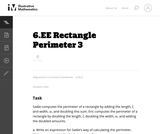Unrestricted Use
CC BY
Rating

The purpose of this task is to ask students to write expressions and to consider what it means for two expressions to be equivalent.

Subject:
Mathematics
Material Type:
Activity/Lab
Provider:
Illustrative Mathematics
Provider Set:
Illustrative Mathematics
Author:
Illustrative Mathematics
08/07/2012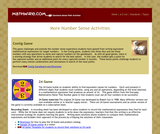Rating

Game 1: Contig Game: This game challenges and extends the number sense experience students have gained from writing equivalent mathematical expressions for target numbers. Game 2: The 24 Game builds on students' ability to find equivalent names for numbers. Game 3: In Tribulation, students must search the game-board for 3 numbers in a row (vertically, horizontally or diagonally as in a word search) that combine to make the target number. In this game, however, there is a prescribed formula for combining the numbers. Students must multiply the first two numbers then add or subtract the third number to produce the target number.

Material Type:
Activity/Lab
Game
Provider:
Mathwire
Author:
Terry Kawas
02/16/2011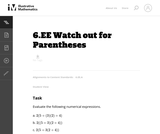Unrestricted Use
CC BY
Rating

This problem asks the student to evaluate three numerical expressions that contain the same integers yet have differing results due to placement of parentheses.

Subject:
Mathematics
Material Type:
Activity/Lab
Provider:
Illustrative Mathematics
Provider Set:
Illustrative Mathematics
Author:
Illustrative Mathematics